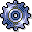Variance CalculationUse

Variances in the Controlling component (CO) at period-end closing can be due to several causes:

• Planning was overshot/undershot
• The actual costs on the cost center or business process differ from the target costs
• Over-/under-absorption occurs on the cost center or the business process

Variance calculation lets you analyze the causes of these variations.

Variance calculation is based on the reconciled planning of internal activity between cost centers and business processes and the costs thereby incurred. Variances are the differences between actual costs and plan costs or target costs. They are displayed separately for a cost center, for an activity type of a cost center or business process, divided into fixed and variable portions. Where possible, they are classified by cost element.

Variance calculation distinguishes between cost centers with activity types (such as production cost centers) and those without (such as administrative cost centers). Actual costs are always posted as activity-independent. To determine the activity input, you therefore need to split the actual costs and the activity-independent plan or target costs of cost centers with activity types on the activity types (see: Actual Cost Splitting). In this way, you can analyze the reasons for the variances for a given cost center activity. Variance calculations compare detailed planning on the activity type level with the corresponding actual costs.

In order to compare plan with actual costs, you must determine the actual activity produced by the cost center or business process in contrast with planning. Variance calculation is therefore carried out on the basis of target costs.

Variance calculation allows you to analyze the actual balance. The system determines the variances of the target costs from the actual costs split on the activity types, as well as from the allocated actual costs in the different variance categories by cost element for each cost center/activity type or business process.

Cost center or business process variances can result from the following situations:

Input and Output Side

These causes can occur separately or together, meaning that variances can originate on both the input and output side of a cost center or business process (see: Variance Categories).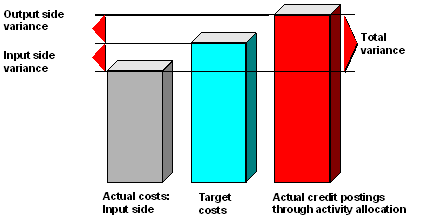Variance Causes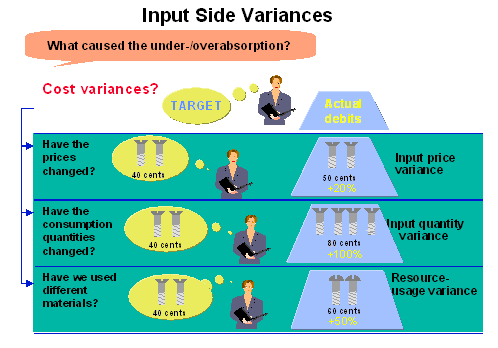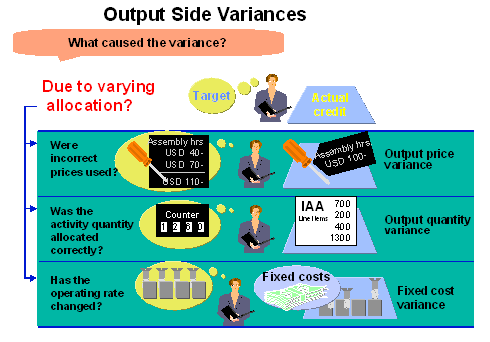Features

Variance calculation is one of the functions performed at period-end closing. Variance calculation uses all the values resulting from all transactions in the Cost Center Accounting (CO-OM-CCA) and Activity-Based Costing components (CO-OM-ABC).

The SAP R/3 System

• Calculates the target costs first
• Splits the actual costs on the activity types
• Calculates variances by cost center, activity type, or business process based on the data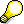In special periods, variances are calculated on the basis of the target or plan costs of the prior periods. This means that in special periods variance calculation can only be carried out cumulatively. To do this, the system imports, the plan and targets costs of the special period in addition to the actual costs, as well as the actual costs of the prior periods. These costs are used to recalculate the overall variances of the analysis period.

You cannot allocate variances further within the Cost Center Accounting or Activity-Based Costing components. You can only credit a cost center or business process completely by using actual price calculation or through settlement to Profitability Analysis (CO-PA).

You can use reporting tools to further analyze the variance calculation results. These means that you can display relevant data divided into fixed and variable portions, or as totals:

In addition, the R/3 System also displays:

• The calculation basis for the individual values (for instance, the distribution base for actual cost splitting)
• How the individual values are made up (for example, individual variance categories)

You can also use the functions Splitting explained, Target costs explained and Variance explained to call up the results in different formats.

As well as changing the list formats, you can also use the totaling and sorting functions. There is also a detail display by cost element for individual objects.

The individual processing steps can be followed successively and you can choose F1 to call up information for the given values.

You can display an overview of the different variance categories in a hierarchical structure. To do so, choose Variance explanation and then Variance categories. You can branch move from this tree structure to the individual variance categories or to the online help. The list for a variance category displays the variances and the relevant formula used for calculating the variance.

Actions

To start variance calculation:

1. Choose Actual postings ® Period-end closing ® Variances
2. Select Cost center, Cost center group, or All cost centers (in Cost Center Accounting) or Business process, Business process group, or All business processes (in Activity-Based Costing), and enter the appropriate object.
3. Enter the period and fiscal year.
4. Select one or more of the following processing indicators.

- Background processing

- Test run

- Detail list

For more information about variance calculation and the functions of the explanation tool, see: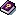Calculating Variances.